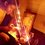# Subtracting Till Infinity

When we have to Calculate a Sum Like this : $\ S=1+\dfrac12+\dfrac14+\dfrac18+\dfrac1{16}+\cdots\infty$

we just multiple by $-\dfrac12$ and Then add the two equations to get our answer , but the same process is not allowed for other series , for example ,

$\text{Let S =}1+3+3^2+3^3+3^4+3^5+\cdots\infty \\ -3S=-3-3^2-3^3-3^4-3^5-\cdots\infty \\ \implies -2S=1 \implies S=-\dfrac12$

why are these type of results considered incorrect ?? Everything i did was correct .

on the other hand sum of series like $\displaystyle\sum_{n=0}^{\infty}2^n=-1$ and $\displaystyle\sum_{n=0}^{\infty}n=-\dfrac1{12}$ are absolutely correct .Note by Sabhrant Sachan
4 years, 2 months ago

This discussion board is a place to discuss our Daily Challenges and the math and science related to those challenges. Explanations are more than just a solution — they should explain the steps and thinking strategies that you used to obtain the solution. Comments should further the discussion of math and science.

When posting on Brilliant:

• Use the emojis to react to an explanation, whether you're congratulating a job well done , or just really confused .
• Ask specific questions about the challenge or the steps in somebody's explanation. Well-posed questions can add a lot to the discussion, but posting "I don't understand!" doesn't help anyone.
• Try to contribute something new to the discussion, whether it is an extension, generalization or other idea related to the challenge.

MarkdownAppears as
*italics* or _italics_ italics
**bold** or __bold__ bold
- bulleted- list
• bulleted
• list
1. numbered2. list
1. numbered
2. list
Note: you must add a full line of space before and after lists for them to show up correctly
paragraph 1paragraph 2

paragraph 1

paragraph 2

[example link](https://brilliant.org)example link
> This is a quote
This is a quote
    # I indented these lines
# 4 spaces, and now they show
# up as a code block.

print "hello world"
# I indented these lines
# 4 spaces, and now they show
# up as a code block.

print "hello world"
MathAppears as
Remember to wrap math in $$ ... $$ or $ ... $ to ensure proper formatting.
2 \times 3 $2 \times 3$
2^{34} $2^{34}$
a_{i-1} $a_{i-1}$
\frac{2}{3} $\frac{2}{3}$
\sqrt{2} $\sqrt{2}$
\sum_{i=1}^3 $\sum_{i=1}^3$
\sin \theta $\sin \theta$
\boxed{123} $\boxed{123}$

Sort by:

the 3 latter sums all diverge,so this value isn't the "true" value of them,meaning that we only assign the series that value,but in the actual sense of equity,this makes no sense.You can read about ways for assigning values to divergent series here

- 4 years, 2 months ago

The series are divergent ,but the sum of the Last two Series are absolutely correct . In this way why can't we say that sum of other infinite [ divergent ]series will also be negative ?

- 4 years, 2 months ago

no,the last 2 divergent series' values are as valid as the value which you gave the 2nd (divergent) series.We can assign that series a negative value,just like the other ones,but that doesn't mean the series doesn't diverge like the others.

- 4 years, 2 months ago

Then what is the significance of these sums if they are all meaningless ?

- 4 years, 2 months ago

they're not meaningless,but you need to be very careful with substituting that value.These series often come up in physics,you can read about it here

Here you can see an example of the series $1+2+3..$ in physics

- 4 years, 2 months ago

This may explain your question: Ramanujan: Making sense of 1+2+3+... = -1/12 and Co.

It talks about this kind of geometry sequences. You may want to skip the 1+2+3+... = -1/12 part.

- 4 years, 2 months ago